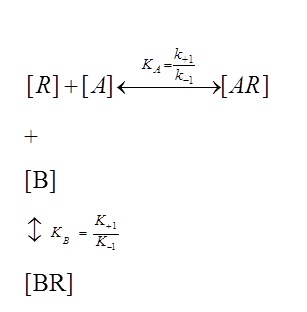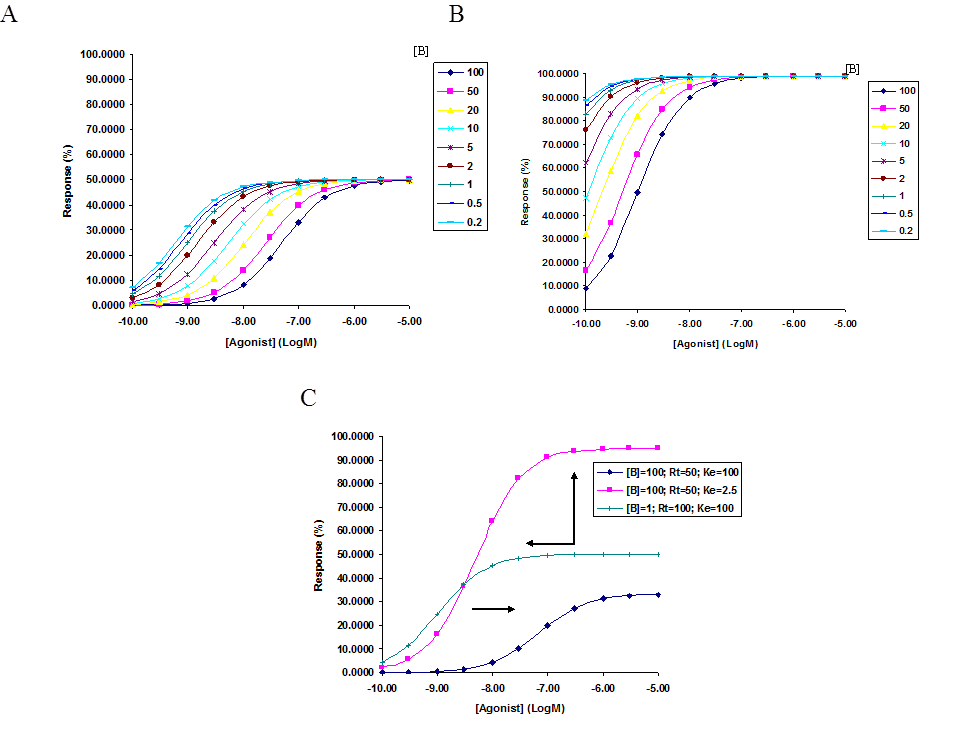# Gaddum modification of Operational Model of Efficacy

The effect of an antagonist on the efficacy of an agonist is based on the following equilibrium relationship wherein the empty receptor (R) competes with the antagonist (B) and the receptor can exist in an antagonist bound state (BR) as well:When applying the law of mass action to these simultaneous equations, we can derive the following relationship:$[AR] = \frac {[A][R_t]}{K_A(1 + \frac {[B]}{K_B]}) + [A]}$                                (1.40)

This equation was derived by J.H. Gaddum (Gaddum 1936) who was the first to modify the Hill-Langmuir equation to incorporate the presence of an antagonist [B]. In this equation,$K_A$ and$K_B$ are the dissociation equilibrium constants for the binding of agonist and antagonist, respectively.

By substituting in the value of [AR] into equation 1.30, we can derive a relationship between the Black and Leff Operational Model of efficacy and occupancy in the presence of a functional antagonist (B) as follows:$\frac{E}{E_m} = \frac{\frac{[A][R_t]}{K_A (1 + \frac {[B]}{K_B}) + [A]}}{K_E + \frac{[A][R_t]}{K_A(1 + \frac{[B]}{K_B}) + [A]}}$ or$\frac{\frac{[A][R_t]}{\frac{K_A K_B + K_A[B] + K_B[A]}{K_B}}}{K_E + \frac{[A][R_t]}{\frac{(K_A K_B + K_A[B] + K_B[A])}{K_B}}}$

This can be further simplified to,$\frac{E}{E_m} = \frac {[A][R_t]K_B}{K_E K_A K_B + K_E K_A[B] + K_E K_B [A] + [A][R_t]K_B}$

Dividing the numerator and the denominator by$K_E$ gives us,$\frac{E}{E_m} = \frac {[A]\tau K_B}{K_A K_B + K_A [B] + K_B [A] + [A] \tau K_B}$

Upon further simplification, this yields the following equation:$E = \frac {E_m \tau [A]}{K_A (1 + \frac{[B]}{K_B} + \frac {[A]}{K_A}) + \tau [A]}$                                  (1.41)

Assuming that the response of a system by an agonist occurs via the same mechanism in the presence or absence of an antagonist and with the assumption that the antagonist is a reversible competitive antagonist, this equation predicts that in the presence of an antagonist, the log agonist concentration response curve is right shifted in parallel (Figure 2). In the absence of an antagonist, where [B] = 0, this equation takes on the form of the Black and Leff Operational Model of Efficacy (equation 1.32). The magnitude of the right shift can be determined similarly to the methods used by H.O. Schild (Schild 1947) in 1949 using the del Castillo-Katz scheme.

We can define equilibrium between two receptor states, in the presence and absence of the antagonist where the degree of receptor occupancy to elicit the same level of response is the same, as follows:$\frac {E_m \tau [A]}{K_A + [A] + \tau [A]} = \frac {E_m \tau r [A]}{K_A (1 + \frac {[B]}{K_B} + \frac {r [A]}{K_A}) + \tau r [A]}$

Here, r is defined as the factor by which the agonist concentration must be adjusted in order to restore a desired response whilst in the presence of an antagonist.The above equation can be further simplified as follows:$\frac {E_m \tau [A]}{K_A + [A] + \tau [A]} = \frac {E_m \tau r [A]}{K_A + r [A] + \tau r [A] + \frac {K_A [B]}{K_B}}$$\frac {E_m \tau [A]}{K_A + [A] + \tau [A]} = \frac {E_m \tau r [A]}{r (\frac{K_A}{r} + [A] + \tau [A]) + \frac {K_A [ B]}{K_B}}$

Dividing the right hand side, numerator and denominator by r, gives,$\frac {E_m \tau [A]}{K_A + [A] + \tau [A]} = \frac {E_m \tau [A]}{(\frac{K_A}{r} + [A] + \tau [A]) + \frac {K_A [B]}{r K_B}}$

Upon cross multiplication, we get$\frac {K_A}{r} + \frac {K_A [B]}{r K_B} + [A] + \tau [A] = K_A + [A] + \tau [A]$

Or,$\frac {K_A}{r} + \frac {K_A [B]}{r K_B} = K_A$  which gives$r = \frac {K_A K_B + K_A [B]}{K_A K_B}$

This can be further simplified to give the following equality, as predicted by Schild:$r - 1 = \frac {[B]}{K_B}$                                                          (1.42)

The equation predicts that the action of an antagonist can be overcome or surmounted by increasing the concentration of the agonist, wherein the magnitude of the concentration ratio of the agonist is only dependent on the concentration of the antagonist, assuming an orthosteric mode of binding.Figure 2. Concentration response theoretical curves generated, in the presence of increasing concentration of an antagonist B, based on the Black and Leff model of Operational Efficacy. The response is right shifted under increasing concentration of the antagonist [B]. Here the affinity of the agonist, $latexK_A$, and antagonist, $latexK_B$ was fixed at 1µM,$[R_t]$ was fixed at a 100 and antagonist [B] titrated from 100 to 0.2. (A) Simulates the response with a low efficacy agonist, with $latexK_E$ = 100. (B) Simulates response with a high efficacy agonist, with $latexK_E$ = 1. (C) Simulates maximal stimulation by high intrinsic efficacy agonist ($latexK_E$ = 2.5) in a desensitized system ($R_t$ = 50) whilst in the presence of an inhibitory concentration of antagonist B.